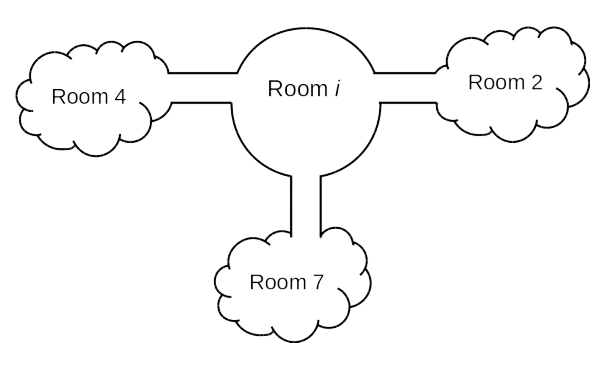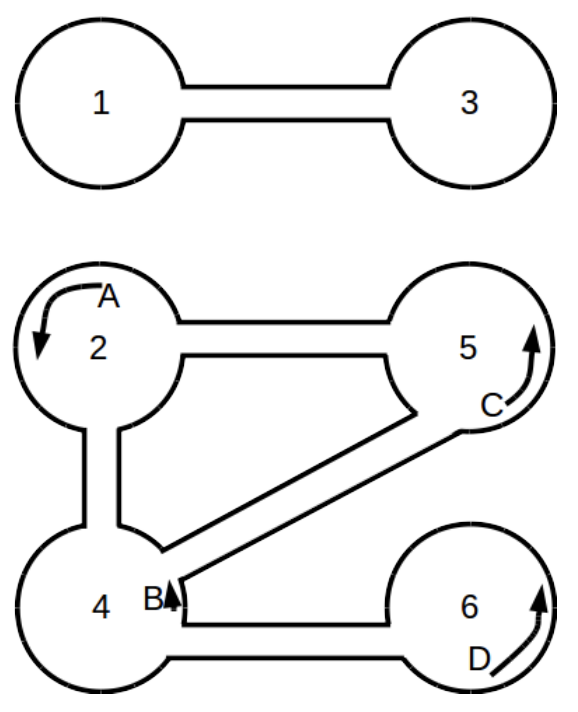시간 제한 메모리 제한 제출 정답 맞은 사람 정답 비율
2 초 512 MB 1 1 1 100.000%

## 문제

There is a maze that is formed by connecting N rooms via some corridors. The rooms are numbered 1 to N and each room has the shape of a circle. The corridors have the following constraints:

• Each corridor forms a connection between two distinct rooms.
• No two corridors will connect the same pair of rooms.
• Each room will have at least 1 corridor that connects to it.

One difficulty in navigating through this maze is that the lights are all out, so you cannot see where you are.

One way to move through the maze is to place your right hand at some point on the wall of the starting room and walk forward through the corridors and other rooms without ever taking your hand off the wall. If you walk through the maze in this way, you will end up returning to the original room. The path that you follow will depend upon where you place your right hand in the starting room, since that will determine which corridor you take first.

Given the structure of the maze you need to answer Q queries. Each query will specify a distinct starting room r. Determine the maximum number of traversals of corridors as you move through the maze by keeping your right hand on the wall beginning in room r and returning (for the first time) to room r.

## 입력

The first line of input contains the integer N(2 ≤ N ≤ 100000).

The next N lines describe the maze’s layout, with the ith of these lines containing information about room i. Specifically, it contains an integer k, the number of corridors connecting to room i, followed by k integers, c1 c2 . . . ck, indicating the rooms that these k corridors lead to (in clockwise order from room i).

For example, if the corridor information for room i is 3 4 2 7, this means that there are 3 corridors connecting room i to rooms 4, 2, and 7. Since they are listed in clockwise order, we would have the following picture:The next line contains an integer Q.

The final Q lines describe the starting rooms to be queried, with each line containing an integer r (1 ≤ r ≤ N).

Let M be the total number of corridors that exist in the maze. The value of M can be calculated as 1/2 (sum of all k values).

## 출력

Output Q lines answering the queries in order.

## 예제 입력 1

6
1 3
2 5 4
1 1
3 2 5 6
2 4 2
1 4
6
1
2
3
4
5
6


## 예제 출력 1

2
5
2
3
5
5


The following picture corresponds to one arrangement of the sample input.Rooms 1 and 3 are connected by one corridor, and it is traversed twice while leaving and returning to the same room, regardless of where we start in rooms 1 or 3.

For room 2, we start at the position A and move counter-clockwise, we will go into the corridors connecting (2, 4), then the corridor connecting (4, 6), then the corridor connecting (6, 4), then the corridor connecting (4, 5), then finally the corridor connecting (5, 2), for a total of 5 traversals of corridors. This is the maximum possible number of traversals.

For room 4, we may start at position B, move counter clockwise, and then traverse corridors (4, 5), (5, 2) and (2, 4), for a total of 3 traversals of corridors. Notice that starting anywhere else in room 4 will yield no more than 3 traversals of corridors.

Starting at positions C and D, in rooms 5 and 6 respectively will yield a reordering of the same path traversed as we found starting from A in room 2, which is yields a total of 5 traversals of corridors in these cases.